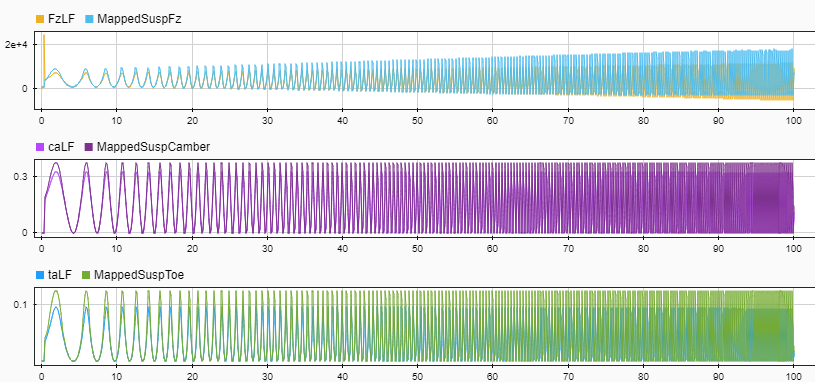## Kinematics and Compliance Virtual Test Laboratory

Model-Based Calibration Toolbox™ allows you to generate optimized suspension parameters for the Independent Suspension - Mapped and Solid Axle Suspension - Mapped blocks by using the kinematics (K) and compliance (C) virtual test laboratory.

To create and open a working copy of the K and C virtual test laboratory reference application, enter

``vdynblksKandCTestLabStart` `

The K and C virtual test laboratory contains vehicle, test system, and test control subsystems. The vehicle system has two variants:

• `Simscape Multibody Vehicle` — Vehicle with a Simscape™ Multibody™ suspension system

• `VDBS Vehicle` — Vehicle with an Independent Suspension - Mapped block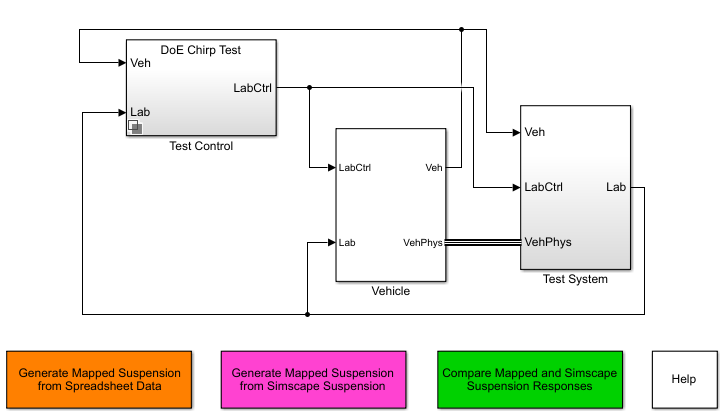This table summarizes the virtual test laboratory tests.

TestObjectiveMethod

Generate Mapped Suspension from Spreadsheet Data

Use measured vertical force and suspension geometry data to generate calibrated suspension parameters for the mapped suspension blocks.

Note

You can use a third-party simulation model to generate the measured suspension data.

The virtual test lab uses Model-Based Calibration Toolbox to fit camber angle, toe angle, and vertical force response models for the data. The virtual test lab then uses the response models to generate suspension parameters for the suspension blocks.

Generate Mapped Suspension from Simscape Suspension

Use a Simscape Multibody suspension system to generate calibrated suspension parameters for the suspension mapped blocks.

The virtual test lab uses Model-Based Calibration Toolbox to perform a Sobol sequence design of experiments (DoE) on the suspension height and handwheel angle operating points. At each operating point, the reference application stimulates the Simscape Multibody suspension system with a chirp signal over a frequency range of 0.1 to 2 Hz. The virtual test lab then uses the data to fit the suspension vertical force, camber angle, and toe angle with a Gaussian process model (GPM) as a function of the suspension state. Finally, the reference application uses the GPM to generate suspension parameters for the suspension blocks.

Compare Mapped and Simscape Suspension Responses

Compare the mapped suspension with the Simscape Multibody suspension results.

The virtual test laboratory stimulates the Simscape Multibody suspension at one operating point and then compares the response to the mapped suspension.

### Generate Mapped Suspension from Spreadsheet Data

The virtual test lab uses Model-Based Calibration Toolbox to fit camber angle, toe angle, and vertical force response models for the data. The virtual test lab then uses the response models to generate suspension parameters for the suspension blocks.

#### Generate Mapped Suspension Calibration

1. Use the Spreadsheet file field to provide a data file. By default, the reference application has `KandCTestData.xlsx` containing required data. The table summarizes the data file requirements for generating calibrated tables.

DataDescriptionData Requirements for Generating Mapped Suspension Tables
z

Vertical axis suspension height, in m

Required

zdot

Vertical axis suspension height velocity breakpoints, in m/s

Required

str

Steering angle, in rad

Required

Fz

Vertical axis suspension force, in N

Required

ca

Camber angle, in rad

Required

ta

Toe angle, in rad

Required

2. Click Generate mapped suspension calibration to generate response surface models in Model-Based Calibration Toolbox.

The model browser opens when the process completes. You can view the camber angle, ca, toe angle, ta, and vertical force, Fz, response model fits for the data.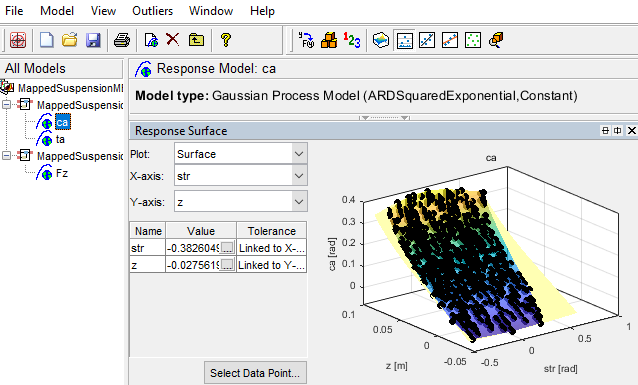#### Apply Calibration to Mapped Suspension Model

1. Click Apply calibration to mapped suspension model. The virtual test lab uses the response models to generate calibrated suspension and breakpoint data.

2. Click OK to update the model workspace and suspension blocks.

In the Model Explorer, you can view the generated suspension parameters.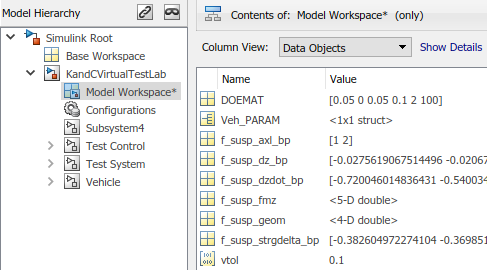ParameterModel Workspace VariableDescription

Axle breakpoints, f_susp_axl_bp

`f_susp_axl_bp`

Axle breakpoints, P, dimensionless.

Vertical axis suspension height breakpoints, f_susp_dz_bp

`f_susp_dz_bp`

Vertical axis suspension height breakpoints, M, in m.

Vertical axis suspension height velocity breakpoints, f_susp_dzdot_bp

`f_susp_dzdot_bp`

Vertical axis suspension height velocity breakpoints, N, in m/s.

Vertical axis suspension force and moment responses, f_susp_fmz

`f_susp_fmz`

`M`-by-`N`-by-`O`-by-`P`-by-`4` array of output values as a function of:

• Vertical suspension height, M

• Vertical suspension height velocity, N

• Steering angle, O

• Axle, P

• 4 output types

• 1 — Vertical force, in N·m

• 2 — User-defined

• 3 — Stored energy, in J

• 4 — Absorbed power, in W

Suspension geometry responses, f_susp_geom

`f_susp_geom`

`M`-by-`O`-by-`P`-by-`3` array of geometric suspension values as a function of:

• Vertical suspension height, M

• Steering angle, O

• Axle, P

• 3 output types

• 1 — Camber angle, in rad

• 2 — Caster angle, in rad

• 3 — Toe angle, in rad

Steering angle breakpoints, f_susp_strgdelta_bp

`f_susp_strgdelta_bp`

Steering angle breakpoints, O, in rad.

### Generate Mapped Suspension from Simscape Suspension

The virtual test lab uses Model-Based Calibration Toolbox to perform a Sobol sequence design of experiments (DoE) on the suspension height and handwheel angle operating points. At each operating point, the reference application stimulates the Simscape Multibody suspension system with a chirp signal over a frequency range of 0.1 to 2 Hz. The virtual test lab then uses the data to fit the suspension vertical force, camber angle, and toe angle with a Gaussian process model (GPM) as a function of the suspension state. Finally, the reference application uses the GPM to generate suspension parameters for the suspension blocks.

The test laboratory exercises the suspension system with the DOE settings contained in the `DOEMAT` array. To view the `DOEMAT` array values, open the Model Explorer.

ElementDescription

`DOEMAT(1,1)`

Suspension height

`DOEMAT(1,2)`

Handwheel angle

`DOEMAT(1,3)`

Chirp signal amplitude

`DOEMAT(1,4)`

Starting chirp frequency

`DOEMAT(1,5)`

Ending chirp frequency

`DOEMAT(1,6)`

Simulation time to complete chirp signal frequency range

The generation can take time to run and slow other computer processes. View progress in the MATLAB® window.

In the Model Explorer, you can view the generated suspension parameters.ParameterModel Workspace VariableDescription

Axle breakpoints, f_susp_axl_bp

`f_susp_axl_bp`

Axle breakpoints, P, dimensionless.

Vertical axis suspension height breakpoints, f_susp_dz_bp

`f_susp_dz_bp`

Vertical axis suspension height breakpoints, M, in m.

Vertical axis suspension height velocity breakpoints, f_susp_dzdot_bp

`f_susp_dzdot_bp`

Vertical axis suspension height velocity breakpoints, N, in m/s.

Vertical axis suspension force and moment responses, f_susp_fmz

`f_susp_fmz`

`M`-by-`N`-by-`O`-by-`P`-by-`4` array of output values as a function of:

• Vertical suspension height, M

• Vertical suspension height velocity, N

• Steering angle, O

• Axle, P

• 4 output types

• 1 — Vertical force, in N·m

• 2 — User-defined

• 3 — Stored energy, in J

• 4 — Absorbed power, in W

Suspension geometry responses, f_susp_geom

`f_susp_geom`

`M`-by-`O`-by-`P`-by-`3` array of geometric suspension values as a function of:

• Vertical suspension height, M

• Steering angle, O

• Axle, P

• 3 output types

• 1 — Camber angle, in rad

• 2 — Caster angle, in rad

• 3 — Toe angle, in rad

Steering angle breakpoints, f_susp_strgdelta_bp

`f_susp_strgdelta_bp`

Steering angle breakpoints, O, in rad.

### Compare Mapped and Simscape Suspension Responses

The virtual test laboratory stimulates the Simscape Multibody suspension at one operating point and then compares the response to the mapped suspension.

• To stimulate the Simscape Multibody suspension model, the test laboratory uses with the DOE settings contained in the `DOEMAT` array.

During the simulation, to view the suspension system, select the tab.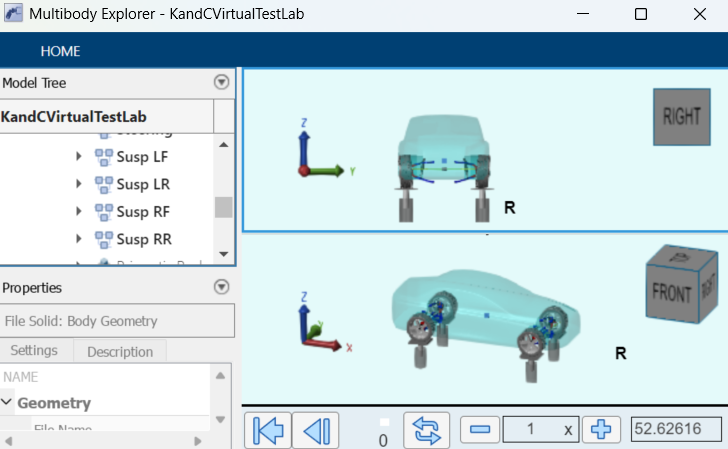• After the simulation completes, use the Simulation Data Inspector to compare the suspension system response for the mapped suspension and Simscape Multibody suspension model. For example, compare the vertical force, camber angle, and toe angle responses.